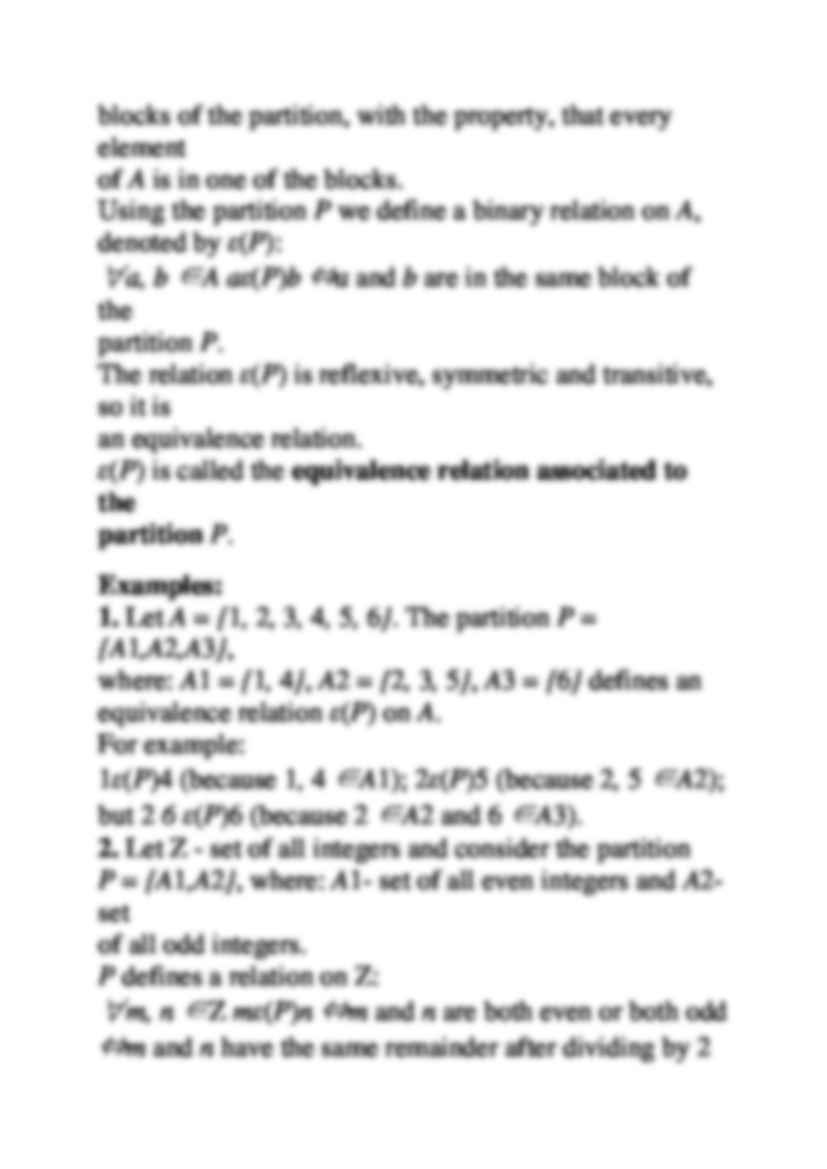# Relacja równoważności - Equivalence relation

Nasza ocena:

Wyświetleń: 707
Komentarze: 0

### Pobierz ten dokument za darmo

Podgląd dokumentu## Fragment notatki:

Equivalence relation Definition Let A be a nonempty set. A binary relation E on A that is reflexive, symmetric and transitive is called an equiva- lence relation on A . If a E b we say, that a is equivalent to b (in sense of relation E ). Examples: 1. The equality relation on a set A is an equivalence relation. 2. The relation of logical equivalence is an equivalence relation on the set Stat ( P ) . 3. Let P be an alphabet. The relation ”has the same length” is an equivalence relation on the set P ⋆ . 4. The relation of congruence modulo k is an equivalence relation on the set Z of all integers. 5. Let L be a set of all straight lines in the plane. The relation l 1 R l 2 ⇔ l 1 || l 2 ( l 1 is parallel to l 2 ) is an equivalence relation on the set L . Let A be a nonempty set and P is a partition of A - a collection of disjoint, nonempty subsets of A , called the blocks of the partition, with the property, that every element of A is in one of the blocks. Using the partition P we define a binary relation on A , denoted by ε ( P ) : ∀ a, b ∈ A a ε ( P ) b ⇔ a and b are in the same block of the partition P . The relation ε ( P ) is reflexive, symmetric and transitive, so it is an equivalence relation. ε ( P ) is called the equivalence relation associated to the partition P . Examples: 1. Let A = { 1 , 2 , 3 , 4 , 5 , 6 } . The partition P = { A 1 ,A 2 ,A 3 } , where: A 1 = { 1 , 4 } , A 2 = { 2 , 3 , 5 } , A 3 = { 6 } defines an equivalence relation ε ( P ) on A . For example: 1 ε ( P )4 (because 1 , 4 ∈ A 1 ); 2 ε ( P )5 (because 2 , 5 ∈ A 2 ); but 2 6 ε ( P )6 (because 2 ∈ A 2 and 6 ∈ A 3 ). 2. Let Z - set of all integers and consider the partition P = { A 1 ,A 2 } , where: A 1 - set of all even integers and A 2 - set of all odd integers. P defines a relation on Z : ∀ m, n ∈ Z m ε ( P ) n ⇔ m and n are both even or both odd ⇔ m and n have the same remainder after dividing by 2 ⇔ 2 | ( m − n ) ⇔ m ≡ n ( mod 2) . P defines the relation of congruence modulo 2. Examples: 3. Consider the partition P = { A 1 ,A 2 ,A 3 } of Z , where A 1 is the set of all integers that are divisible by 3: A 1 = { ..., − 9 , − 6 , − 3 , 0 , 3 , 6 , 9 , ... ... zobacz całą notatkę

## Komentarze użytkowników (0)

Zaloguj się, aby dodać komentarz